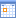Find Order details

 Find Order details Idorder is equal tois not equal tois less thanis less than or equal tois greater thanis greater than or equal to Name containsstarts withends withis equal tois not equal tois less thanis less than or equal tois greater thanis greater than or equal to Telephone is equal tois not equal tois less thanis less than or equal tois greater thanis greater than or equal to Product containsstarts withends withis equal tois not equal tois less thanis less than or equal tois greater thanis greater than or equal to Price quote is equal tois not equal tois less thanis less than or equal tois greater thanis greater than or equal to Supplier containsstarts withends withis equal tois not equal tois less thanis less than or equal tois greater thanis greater than or equal to Date ordered is equal tois not equal tois less thanis less than or equal tois greater thanis greater than or equal toCost is equal tois not equal tois less thanis less than or equal tois greater thanis greater than or equal to Date delivered is equal tois not equal tois less thanis less than or equal tois greater thanis greater than or equal toTel customer is equal tois not equal tois less thanis less than or equal tois greater thanis greater than or equal to Collected is equal tois not equal tois less thanis less than or equal tois greater thanis greater than or equal to